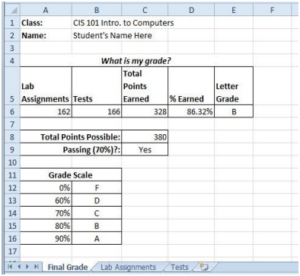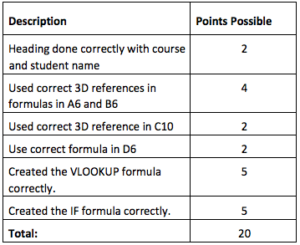### Student Learning Outcomes:

1. Create 3D formulas across multiple worksheets

2. Use the VLOOKUP function

3. Use the IF function

### Preparation: (Review these terms)

• 3-D Reference Information
• VLOOKUP Information
• IF function Information

### New Terms:

• 3-D Referencing used in Step 5 (reference the information sheet)  Calculate grade earned (Step- 9, page 2)
• VLOOKUP (Step 11, page 2)
• VLOOKUP argument box (Step 12, page 2)

### Narrative:

This assignment has many steps. All cells that you will work in must have formulas. Hopefully you will give yourself time to complete this exercise. (THIS ASSIGNMENT IS WORTH 20 POINTS. THE RUBRIC IS ON MAKE 3)

2. In cell B1 replace “CIS 101 Intro. to Computers” with the title of your class.

3. In cell B2 replace “Student’s Name Here” with your actual name.

4. Become familiar with the workbook by reviewing the Final Grade, Lab Assignments, and Tests worksheets names found at the bottom of the screen.

5. Create a 3-D Reference in cell A6 in the Final Grade Sheet. You will be referencing the Lab Assignment sheet and choosing the total points earned. (Hint: See 3-D Referencing Instruction Sheet and follow the example.]

6. Repeat the steps above for a 3-D Reference in cell B6 in the Final Grade Sheet. You will be referencing the Test Sheet and the total points earned.

7. In cell C6 use the SUM function to add cells A6 and B6 together.

8. In cell C8 create a formula to calculate the Total Points Possible. (Hint: you are creating another formula that uses 3D references. You need to add the Total Points Possible in cell C2 on the Lab Assignments worksheet to the Total Points Possible in cell C2 in the Tests worksheet. Just use the plus sign between the formula of the sheets.)

9. In D6 create a formula to calculate the % earned. This formula requires the total points earned to be divided by the total points possible for the Lab Assignments and Test sheets.

10. In cell E6 create a formula to look up the letter grade in the Grade Scale table. You will be referencing the table without the title from A11 to B16.

11. Use the VLOOKUP function. (Hint: Use the VLOOKUP instruction sheet. Follow the example on the sheet to make sure you know how to do this formula.)

12. In cell C9 create a formula using the IF function to determine whether the student is passing the class with 70% or better. If the student is passing, the cell should display “Yes”. If the student is not passing, then the cell should display “No”. (Hints: Use the argument box for the IF Function. For more help with this, be sure to find IF Function Instructions in the Extra Help Folder and follow the example.)

13. Print the document if your instructor requires a hard copy or submit it online.

14. Your finished spreadsheet should look like the worksheet below. Please be sure to use formulas based upon the above instructions. Your instructor will check your formulas and take points off if you just type in the results and not a formula.

15. Save your work and submit to instructor

### Completed Worksheet Assignment### Check the rubric below to be sure you have completed all the tasks.